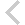FICO Xpress Optimization Examples Repository
 FICO Optimization Community FICO Xpress Optimization HomeFolio - Modelling examples from 'Getting started'

Description
• Chapter 3 Inputting and Solving a Linear Programming problem
• foliolp.mos: modeling and solving a small LP problem
• foliolperr.mos: LP model with syntax errors
• foliolps.mos: LP model using string indices
• Chapter 4 Working with data
• foliodata.mos (data file: folio.dat): data input from file, result output to a file, model parameters
• folioodbc.mos (data files: folio.xls, folio.mdb, folio.sqlite): data input from a spreadsheet or database, result output to a spreadsheet or database, model parameters
• folioexcel.mos (data file: folio.xls): same as folioodbc.mos but with Excel-specific data input and output (Windows only)
• foliosheet.mos (data file: folio.xls): same as folioodbc.mos but with data input and output through generic spreadsheet access
• foliocsv.mos (data file: folio.csv): same as folioodbc.mos but with data input and output through generic spreadsheet access in CSV format
• Chapter 5 Drawing user graphs
• folioloop.mos (data files: folio.dat, foliodev.dat): re-solving with varied parameter settings
• folioloop_graph.mos (data files: folio.dat, foliodev.dat): re-solving with varied parameter settings, graphical solution display
• foliolps_graph.mos: same as foliolps, adding graphical solution display
• Chapter 6 Mixed Integer Programming
• foliomip1.mos (data file: folio.dat): modeling and solving a small MIP problem (binary variables)
• foliomip2.mos (data file: folio.dat): modeling and solving a small MIP problem (semi-continuous variables)
• folioqp.mos (data file: folioqp.dat): modeling and solving a QP and a MIQP problem
• folioqp_graph.mos (data files: folioqp.dat, folioqpgraph.dat): re-solving a QP problem with varied parameter settings, graphical solution display
• folioqc.mos (data file: folioqp.dat): modeling and solving a QCQP and
• foliomiqc.mos (data file: folioqp.dat): modeling and solving a MIQCQP
• Chapter 8 Heuristics
• folioheur.mos (data file: folio.dat): heuristic solution of a MIP problem

Source Files

Data Files

folioqp_graph.mos

```(!******************************************************
Mosel Example Problems
======================

file folioqp_graph.mos
``````````````````````
Modeling a small QP problem
to perform portfolio optimization.
Minimize variance subject to different target return.
Graphical output.

(c) 2008 Fair Isaac Corporation
author: S.Heipcke, Aug. 2003, rev. Sep. 2017
*******************************************************!)

model "Portfolio optimization with QP, graphical output"
uses "mmxprs", "mmnl", "mmsvg"      ! Use Xpress Optimizer with QP solver

parameters
MAXVAL = 0.3                       ! Max. investment per share
MINAM = 0.5                        ! Min. investment into N.-American values
end-parameters

declarations
SHARES = 1..10                     ! Set of shares
RISK: set of integer               ! Set of high-risk values among shares
NA: set of integer                 ! Set of shares issued in N.-America
RET: array(SHARES) of real         ! Estimated return in investment
VAR: array(SHARES,SHARES) of real  ! Variance/covariance matrix of
! estimated returns
SOLRET: array(range) of real       ! Solution values (total return)
SOLDEV: array(range) of real       ! Solution values (average deviation)
end-declarations

initializations from "folioqp.dat"
RISK RET NA VAR
end-initializations

declarations
frac: array(SHARES) of mpvar       ! Fraction of capital used per share
end-declarations

! Objective: mean variance
Variance:= sum(s,t in SHARES) VAR(s,t)*frac(s)*frac(t)

! Minimum amount of North-American values
sum(s in NA) frac(s) >= MINAM

! Spend all the capital
sum(s in SHARES) frac(s) = 1

! Upper bounds on the investment per share
forall(s in SHARES) frac(s) <= MAXVAL

! Solve the problem for a range of returns: this is the efficient frontier
target:= min(s in SHARES) RET(s)
RMAX:= max(s in SHARES) RET(s)

while(target < RMAX) do
Return:= sum(s in SHARES) RET(s)*frac(s) >= target    ! Target yield
minimize(Variance)                ! Solve the problem

if (getprobstat = XPRS_OPT) then  ! Save the optimal solution value
ct+=1
SOLDEV(ct):= getobjval
SOLRET(ct):= target
else
writeln("No solution for target return >= ", ct, "%")
break
end-if
target += 1
end-do

! Drawing a graph to represent results (`GrS') and data (`GrL' & `GrH')
declarations
DEV: array(SHARES) of real         ! Standard deviation
NAMES: array(SHARES) of string     ! Names of shares
end-declarations

initializations from "folioqpgraph.dat"
DEV NAMES
end-initializations

forall(r in 1..ct) svgaddpoint("GrS", SOLRET(r), SOLDEV(r));

svgaddline("GrS", sum(r in 1..ct) [SOLRET(r), SOLDEV(r)])

forall (s in SHARES-RISK) do
end-do

forall (s in RISK) do
end-do

! Scale the size of the displayed graph
svgsetgraphscale(10)
svgsetgraphpointsize(2)
svgsetgraphlabels("Expected return", "Standard deviation")

! Optionally save graphic to file
svgsave("folioqpgraph.svg")

! Display the graph and wait for window to be closed by the user
svgrefresh
svgwaitclose("Close browser window to terminate model execution.", 1)

end-model

```